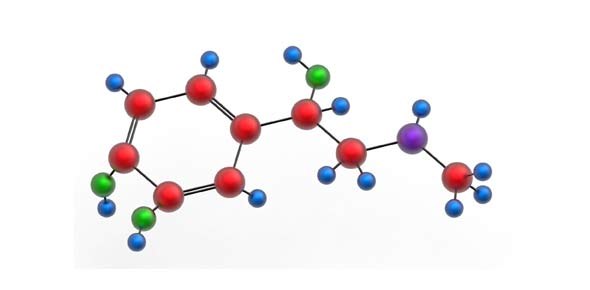# Coordination Compounds

15 Questions | Attempts: 2509
ShareSettingsCoordination complexes in chemistry consist of atoms, which are metallic in nature and an array of boundless ions that are known as complexing agents. Find out about this and more through the quiz below. All the best.

• 1.
When 1 mol of CrCl3.6H2O is treated with excess of AgNO3, 3 mol of AgCl is obtained. The formula of the complex is
• A.

[CrCl3(H2O)3].3H2O

• B.

[CrCl2(H2O)4]Cl.2H2O

• C.

[CrCl(H2O)5]Cl2.H2O

• D.

[Cr(H2O)6]Cl3

• 2.
The compounds [Co(SO4)(NH3)5]Br and [Co(SO4)(NH3)5]Cl represent:
• A.

• B.

Ionisation isomerism

• C.

Coordination isomerism

• D.

No isomerism

• 3.
2,4- Dinitrophenyl hydrazine is an example for:
• A.

Tridentate ligand

• B.

Monodentate ligand

• C.

Polydentate ligand

• D.

Didentate ligand

• 4.
Which of the following complex ion shows geometrical isomerism?
• A.

[Cr(H2O)4Cl2]+

• B.

[Pt(NH3)3Cl]

• C.

[Co(NH3)6]3+

• D.

[Co(CN)5(NC)]3-

• 5.
The oxidation state of Fe in the brown ring complex [Fe(H2O)5NO]SO4 is:
• A.

+ 1

• B.

+ 2

• C.

+ 3

• D.

+ 4

• 6.
Which of the following can exhibit geometrical isomerism?
• A.

[MnBr4]2-

• B.

[Pt(NH3)3Cl]+

• C.

[PtCl2P(C2H5)3]2

• D.

[Fe(H2O)5NO]2+

• 7.
According to IUPAC nomenclature, sodium nitroprusside is named as:
• A.

Sodium nitroferricyanide

• B.

Sodium nitroferrocyanide

• C.

Sodium pentacyano nitrosylferrate (II)

• D.

Sodium pentacyano nitrosylferrate (III)

• 8.
Hybridisation and shape of K3[Co(CO3)3] is:
• A.

D2sp3and Octahedral

• B.

Sp3d2 and Octahedral

• C.

Dsp2 and square planar

• D.

Sp3 and tetrahedral

• 9.
Total number of possible isomers for the complex compound [CuII(NH3)4][PtIICl4] are:
• A.

3

• B.

4

• C.

5

• D.

6

• 10.
Square planar complexes of the type MABXL (where A, B, X and L are unidentates) show:
• A.

Two cis and one trans isomer

• B.

Two trans and one cis isomer

• C.

Two cis and two trans isomer

• D.

One cis and one trans isomer

• 11.
In spectrochemical series, chlorine is above water i.e., Cl > H2O, this is due to:
• A.

Good π-acceptor properties of Cl

• B.

Strong σ-donor and good π-acceptor properties of Cl

• C.

Good π-donor properties of Cl

• D.

Larger size of Cl than H2O

• 12.
Which of the following shall form an octahedral complex?
• A.

D4 (low spin)

• B.

D8 (high spin)

• C.

D6 (low spin)

• D.

All of these

• 13.
Which of the following is diamagnetic in nature?
• A.

Co3+, octahedral complex with weak field ligand

• B.

Co3+, octahedral complex with strong field ligand

• C.

Co2+ in tetrahedral complex

• D.

Co2+ in square planar complex

• 14.
The value of n in the carbonyl: (CO)n – Co – Co – (CO)n
• A.

4

• B.

5

• C.

6

• D.

7

• 15.
If the length of CO bond in carbon monoxide is 1.128 Ǻ, then what is the value of CO bond length in Fe(CO)5?
• A.

1.15 Ǻ

• B.

1.128 Ǻ

• C.

1.72 Ǻ

• D.

1.118 Ǻ

## Related TopicsBack to top
×

Wait!
Here's an interesting quiz for you.